﻿ Formula Operators

# Formula Operators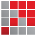When setting up formulae in Accelerus, you may use any of the operators which are available in the Calculation Editor's Operators pane.

These are divided into five branches and many will be familiar, particularly those in the Mathematical and Comparison branches, eg Addition, Subtraction, Greater Than, and so forth.

Others may not be so familiar, but these are not commonly used, eg the Binary operators.

Each branch and the operators within these are outlined below, with examples of each being provided.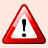When using the various operators, it is important to remember the precedence rules for your operators, ie which take precedence over or are evaluated before others, or your calculated results may be incorrect.Binary Operators

Binary or bitwise operators are specialised operators which deal with the binary values of numbers.

The use of bitwise operators requires a good understanding of binary number systems and low level computer storage.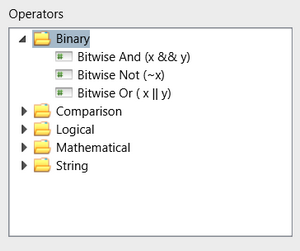Bitwise Not (~ x)
 Action/Description Converts every 0 in a binary number to 1 and every 1 to 0. Because of the way numbers are stored, ~0 equals –1 and ~1 equals –2.Bitwise (x && y)
 Action/Description Looks at each binary digit in the two operands and creates a new number whereby if both operand digits are 1, the new digit is 1, otherwise the new digit is 0. Example syntax {Student.YearLevel} && 2 Example usage/s The above expression will equal 2 for Year Levels 2, 3, 6, 7, 10, 11, and 0 for year levels 1, 4, 5, 8, 9, 12.Bitwise Or (x || y)
 Action/Description Looks at each binary digit in the two operands and creates a new number whereby if both operand digits are 0, the new digit is 0, otherwise the new digit is 1. Example syntax {Student.YearLevel} || 2 Example usage/s For year levels from 1 to 12, the above results will be 3, 2, 3, 6, 7, 6, 7, 10, 11, 10, 11, 14.Comparison Operators

Comparison operators comprise:

 • The six equality operators, eg =, >, <=, etc.
 • The In operator
 • The Like operator

Each comparison operator results in a Boolean value, ie True or False.Equal (x = y)
 Action/Description True if the two operands are identical Example syntax {Student.YearLevel} = 7Not Equal (x <> y)
 Action/Description True if the values for the two operands differ Example syntax {EnteredValue} <> “A+”Less Than (x < y)
 Action/Description True if the first operand x is less than the second operand y Example syntax {EnteredValue} < 50Less Than or Equal (x <= y)
 Action/Description True if the first operand is less than or identical to the second operand Example syntax {EnteredValue} <= 50Greater Than (x > y)
 Action/Description True if the first operand x  is greater than the second operand y Example syntax {Subject.Level} > {Student.YRLV.Code}Greater Than or Equal (x >= y)
 Action/Description True if the first operand is greater than or identical to the second Example syntax {TOTAL.Min} >= 10In (x IN (a,b,c))

Action/Description

True if the first operand x is one of the items in the list

Example syntax

{Subject.Code} IN (“7ENG”, “ENG7”, “ENG7A”)

Example usage/s

Where it is possible to use the equality comparison operators to test for conditions being met, do so. However in some cases it is difficult to see any pattern to test against. In these cases, the In operator allows matching to a specific list.You may use the logical NOT operator in conjunction with the In operator to produce the equivalent of NotIn which can be found in the Analyser's Source and Expand Operators, eg: NOT({Subject.Code} IN (“7ENG”, “ENG7”, “ENG7A”))Like (x LIKE y)

Action/Description

True if the first operand matches the second operand where the second may use wildcard pattern matching.

The pattern matching options are:

*

Matches any set of 0 or more characters.

?

Matches any single alphanumeric character.

#

Matches any single numeric character.

[list]

A list of characters enclosed in square brackets may be used to match against any single character in the list.

[!list]

An exclamation mark followed by a list of characters, all enclosed in square brackets, matches any character not in the list.

[char1-char2]

A hyphen used inside a square bracket character list matches the range of characters.

Example syntax

{Subject.Name} like “*Eng*”

{AI.Code} like “WR”

{Student.FamilyName} like “[QW-Z]*[AEIOU]”

Example usage/s

The Like operator is used where there is some similarity between the set of acceptable values.

In the first example, the similarity is that the characters Eng must exist somewhere in the subject name.

In the second example, for the assessment item code to match, it must be one of WR1, WR2, WR4 or WR7.

In the third example, the student’s family name must start with Q, W, X, Y, or Z and end in a vowel.You may use the logical NOT operator, in conjunction with the Like operator to produce the equivalent of NotLike which can be found in the Analyser's Source and Expand Operators. For example: NOT({Subject.Name} like “*Eng*)Logical Operators

Logical operators work with Boolean values, ie True or False.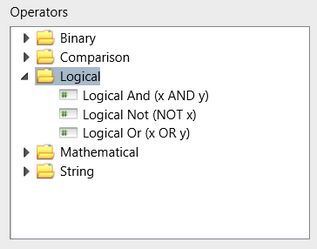Logical Not (NOT x)
 Action/Description Converts true to false and false to true Example syntax NOT ({Student.YRLV.Code} = {Subject.Level})Logical And (x AND y)
 Action/Description If both operands are true, the entire expression is true. If either is false, the result is false. Example syntax {Subject.Cycle.Name} = 2012) AND ({Class.Code} = “10ENGA”)Logical Or (x OR y)
 Action/Description If either operand is true, the expression is true. If both are false, the result false. Example syntax ({Student.GivenName} like “Jo*”) OR ({Student.PreferredName} like “Jo*”) Example usage/s If you knew a student’s name began with “Jo” but didn’t know if that was their given name or preferred name, the above formula would select all students which matched either situation.Mathematical Operators

Mathematical operators consist of the standard addition, subtraction, multiplication and division operators, all of which use two operands.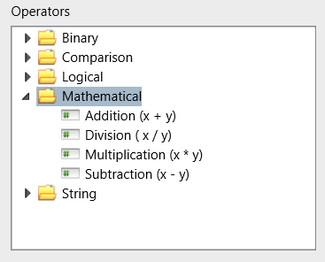Addition (x + y)
 Action/Description Adds the two operands, x and y, together Example syntax {WHAvg1} + {WHAvg2}Division (x / y)
 Action/Description Divides the first operand x by the second y Division by zero is an error Example syntax {TotalPoints} / {SubjCount}Multiplication (x * y)
 Action/Description Multiplies the two operands together Example syntax {SubjAvg} * 100Subtraction (x - y)
 Action/Description Subtracts the second operand y from the first operand x Example syntax {EnteredValue} – 1String Operators

There is only one string operator – Concatenation.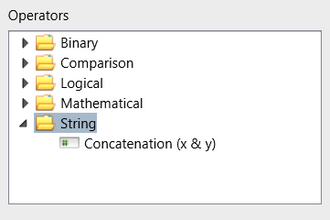Concatenation (x & y)
 Action/Description Joins two text strings together. Example syntax {Subject.Code} & “ – “ & {Subject.Name} Example usage/s Where it is desired that two string fields be joined into a single field, the concatenation operator is used. In the above example, the subject code will be followed by a dash and then the subject name, eg: 07ENG – English 7